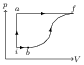# First Law of Thermodynamics and its Applications

## Problems from IIT JEE

Problem (IIT JEE 2014):A thermodynamic system is taken from an initial state i with internal energy $U_\text{i}=100\;\mathrm{J}$ to the final state f along two different paths iaf and ibf, as schematically shown in the figure. The work done by the system along the path af, ib and bf are $W_\text{af}={200}\;\mathrm{J}$, $W_\text{ib}={50}\;\mathrm{J}$ and $W_\text{bf}={100}\;{J}$ respectively. The heat supplied to the system along the path iaf, ib and bf are $Q_\text{iaf}$, $Q_\text{ib}$ and $Q_\text{bf}$ respectively. If the internal energy of the system in the state b is $U_\text{b}={200}\;\mathrm{J}$ and $Q_\text{iaf}={500}\;\mathrm{J}$, the ratio $Q_\text{bf}/Q_\text{ib}$ is,

Solution: In a thermodynamics process, the heat supplied to the system, the increase in internal energy of the system, and the work done by the system are related by the first law of thermodynamics, $\Delta Q=\Delta U+\Delta W$.

The first law of thermodynamics for the process iaf gives, \begin{align} \label{hxb:eqn:1} Q_\text{iaf}=U_\text{iaf}+W_\text{iaf}=(U_\text{f}-U_\text{i})+(W_\text{ia}+ W_\text{af}). \end{align} Substitute $Q_\text{iaf}={500}\mathrm{J}$, $U_\text{i}={100}\;\mathrm{J}$, $W_\text{ia}=0$ (constant volume), and $W_\text{af}={200}\mathrm{J}$ to get $U_\text{f}={400}\;\mathrm{J}$.

In the process ib, \begin{align} \label{hxb:eqn:2} Q_\text{ib}= U_\text{ib}+ W_\text{ib}=(U_\text{b}-U_\text{i})+ W_\text{ib}. \end{align} Substitute $U_\text{b}={200}\;\mathrm{J}$, $U_\text{i}={100}\;\mathrm{J}$, and $W_\text{ib}={50}\;\mathrm{J}$ to get $Q_\text{ib}={150}\;\mathrm{J}$.

In the process bf, \begin{align} \label{hxb:eqn:3} Q_\text{bf}=U_\text{bf}+ W_\text{bf}=(U_f-U_b)+ W_\text{bf}. \end{align} Substitute $U_\text{f}={400}\;\mathrm{J}$, $U_\text{b}={200}\;\mathrm{J}$ and $W_\text{bf}={100}\;\mathrm{J}$ to get $Q_\text{bf}={300}\;\mathrm{J}$. Thus, $Q_\text{bf}/Q_\text{ib}=300/150=2$.

Problem (IIT JEE 2001): In a given process of an ideal gas, $\text{d}W=0$ and $\text{d}Q<0$. Then for the gas,

1. the temperature will decrease.
2. the volume will increase.
3. the pressure will remain constant.
4. the temperature will increase.

Solution: First law of thermodynamics, $\mathrm{d}Q=\mathrm{d}U+\mathrm{d}W$, gives $\mathrm{d}U<0$. For an ideal gas, internal energy decreases due to decrease in temperature.